Test Description

## 15 Questions MCQ Test Olympiad Preparation for Class 10 - Math Olympiad Test: Quadratic Equations- 2

 1 Crore+ students have signed up on EduRev. Have you?

### Find the value of x. (Be sure to give all solutions) x2 - 6 = 138

Detailed Solution for Math Olympiad Test: Quadratic Equations- 2 - Question 1

Simplifying x2 + -6 = 138
Reorder the terms: -6 + x2 = 138
Solving -6 + x2 = 138
Solving for variable 'x'
Move all terms containing x to the left, all other terms to the right
Add '6' to each side of the equation
-6 + 6 + x2 = 138 + 6
Combine like terms: -6 + 6 = 0
0 + x2 = 138 + 6
x2 = 138 + 6
Combine like terms: 138 + 6 = 144
x2 = 144
Simplifying x2 = 144
Take the square root of each side:
x = {-12, 12}

### Find the values for x for the following equation. x2 + 4x - 32 = 0

Detailed Solution for Math Olympiad Test: Quadratic Equations- 2 - Question 2

This equation is written in standard form and it is set equal to 0. Therefore, I know that the 2 methods for solving are factoring and using the quadratic equation.
As I browse the answers, it looks like the values for x are integers, so I must be able to factor. Therefore, this will be the easiest method.
I need to find two integers whose product is -32 and whose sum is 4. (8 & -4).
(x + 8) (x - 4) = 0 Rewrite as factors
x + 8 = 0 x - 4 = 0
Set each factor equal to 0
x + 8 - 8 = 0 - 8
x - 4 + 4 = 0 + 4
x = - 8,  x = 4

### Find the values of y for the following equation: 2y2 - 5y + 2 = 5

Detailed Solution for Math Olympiad Test: Quadratic Equations- 2 - Question 3

In order to find the values for y, we must set the equation equal to 0 by subtracting 5 from both sides.
2y2 - 5y + 2 - 5 = 5 - 5
Subtract 5 from both sides
2y2 - 5y - 3 = 0
Now the equation is set equal to 0.
As I take a look at the answers, it looks like this equation can be factored. Let’s try!
(2y + 1) (y - 3) = 0 Factor the equation
2y + 1 = 0   y - 3 = 0
Set each factor equal to 0
2y + 1 - 1 = 0 - 1     y - 3 + 3 = 0 + 3
2y = -1   y = 3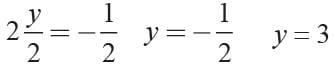A ball is shot from a cannon into the air with an upward velocity of 36 ft/sec. The equation that gives the height (h) of the ball at any time (t) is: h(t) = -16t2 + 36t + 1.5. Find the maximum height attained by the ball.

Detailed Solution for Math Olympiad Test: Quadratic Equations- 2 - Question 4

In order to find the maximum height of the ball, I would need to find the y-coordinate of the vertex.
Vertex formula: x = - b/2a
where: a = -16 b = 36 c = 1.5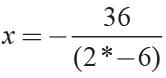x = 1.125
Now substitute 1.125 for t into the equation and solve for h(t). H(t) = -16 (1.125)2 + 36 (1.125) + 1.5
H(t) = 21.75

If a < 0, then function f(x) = ax2 + bx + c has a maximum value at

Detailed Solution for Math Olympiad Test: Quadratic Equations- 2 - Question 5

The graph of a quadratic equation is parabola.
If a < 0, then the parabola opens downward. If the parabola opens downward, then the vertex is the point whose y-value is the maximum value of f.

Detailed Solution for Math Olympiad Test: Quadratic Equations- 2 - Question 6

Quadratic function is a function that can be described by an equation of the form fxx = ax2 + bx + c, where a ≠ 0.
In a quadratic function, the greatest power of the variable is 2. The graph of a quadratic function is a parabola.

If α  and β are the roots of 3x2 + 8x +2 = 0 then what is the value α2 + β2?

Detailed Solution for Math Olympiad Test: Quadratic Equations- 2 - Question 7

Given equation is
3x2 + 8x + 2 = 0
and α, β are its roots then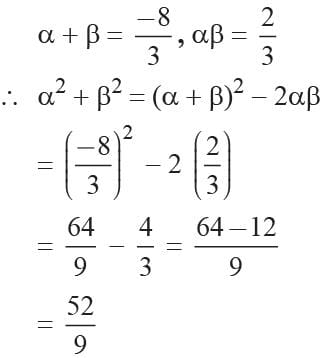If the sum of roots of the equation Kx2 + 2x + 3K = 0 is equal to their product, then the value of K is

Detailed Solution for Math Olympiad Test: Quadratic Equations- 2 - Question 8

Here Kx2 + 2x + 3K = 0
then given Sum of roots = Product of roots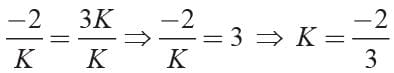The roots of a quadratic equation are 7 and -3, then what is the equation?

Detailed Solution for Math Olympiad Test: Quadratic Equations- 2 - Question 9

The required equation is
x2 - (Sum of roots)x + Product of roots = 0
⇒ x2 - [7 + (- 3)]x + (7) (- 3) = 0
⇒ x2 - 4x - 21 = 0

If one root of the equation x2 + px + 12 = 0 is 4, while the equation x2 + px + q = 0 has equal roots then the value of q is

Detailed Solution for Math Olympiad Test: Quadratic Equations- 2 - Question 10

Here x2 + px + q = 0 has one root a = 4
∴ 42 + b4 + 12 = 0
⇒ b = -7
Now the equation x2 - 7x + q = 0 has equal roots
(-7)2 - 4 (1) (q) = 0 then
⇒ 4q = 49 ⇒ q = 49/4

If α , β are the roots of the equation x2 - p (x + 1) - c = 0 then (α + 1) (β + 1) is equal to

Detailed Solution for Math Olympiad Test: Quadratic Equations- 2 - Question 11

The given equation is
x2 - p(x + 1) - c = 0
⇒ x2 - px + (-c - p) = 0
∴ α + β = p
and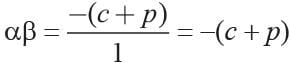Now (α + 1) (β+ 1) = αβ + α + β + 1 = - c -p + p + 1 = 1 - c

If the difference of the root x2 -px + q = 0 is unity then

Detailed Solution for Math Olympiad Test: Quadratic Equations- 2 - Question 12

We have x2 - px + q = 0
Now α + β = p, αβ = q
∴ α - β = 1
2α = p + 1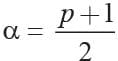Now
β = p - α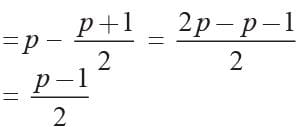and     αβ = q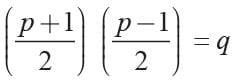p2 - 1 = 4q ⇒ p2 - 4q = 1

If a, b are the roots of the equation x2 + x + 1 = 0 then a2 + b2 = ?

Detailed Solution for Math Olympiad Test: Quadratic Equations- 2 - Question 13

Given a and b are roots of x2 + x + 1 = 0
then a + b = -1 and ab = 1
∴ a2 + b2 = (a + b)2 - 2ab
= (-1)2 - 2(1) = 1 - 2 = -1

If A and B are the roots of the quadratic equation x2 - 12x + 27 = 0, then A3 + B3 is

Detailed Solution for Math Olympiad Test: Quadratic Equations- 2 - Question 14

Given A and B are the roots of x2 - 12x + 27 = 0
⇒ (x - 3)(x - 9) = 0
∴ A =  3, B = 9
Hence A3 + B3 = 33 + 93 = 27 + 729 = 756

If the quadratic equation (a2 - b2)x2 + (b2 - c2)x + (c2 - a2) = 0 has equal roots, then which of the following is true?

Detailed Solution for Math Olympiad Test: Quadratic Equations- 2 - Question 15

If the quadratic equation is given by Ax2 + Bx + C = 0.
Then,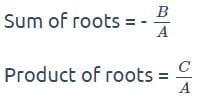Given: (a2 - b2)x2 + (b2 - c2)x + (c2 - a2) = 0
Where,
A = (a2 - b2), B = (b2 - c2), C = c2 - a2
Calculation:
Here,
A + B + C = (a2 - b2) + (b2 - c2) + (c2 - a2) = 0.
So, we can say that x = 1 is a root of a given quadratic equation. According to question both roots are same.
Then,
Roots (x) = 1, 1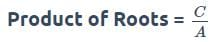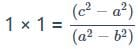(a2 - b2) = (c- a2)
By arranging, we get
b2 + c2 = 2a2

## Olympiad Preparation for Class 10

11 videos|36 docs|201 tests